## Part 1. Statistical terms

#### Sample and Population

Statistics as a scientific method is used for studying properties of the target population by using observed data from a random sample from the target population. The idea is that we can do informative conclusions about some properties of the whole population based on a relatively small subsample from that population, as long as the sampling and conclusions are made in an appropriate way.

#### Examples of uses of statistics

• We want to study cholesterol levels among the target population of 40-50 years old Finnish residents. There are over 500,000 people in this target population and, for various reasons, we cannot get access to the cholesterol levels of all of them. Instead, we can access a random subsample of size 1,000 individuals from the target population collected by the FinHealth study, and we can study their cholesterol value distribution. As we will see later on this course, statistics tells us how accurately we are estimating the mean cholesterol level in the whole target population by using a random subsample of 1,000 individuals from that population.

• We want to study whether a candidate drug is efficient against chronic migraine. Our target population is patients with chronic migraine. Again, we cannot try the candidate drug on all patients for various reasons. More feasibly, we can put up a clinical trial where we recruit 200 patients, randomly split them into two equal sized groups and treat one group (treatment group) with the candidate drug and the other group (control group) with the current standard treatment. We measure which proportion of patients in each group has benefited from their treatment. Then we do statistics to see whether the proportions in the two groups seem to say that the candidate drug works clearly better in the target population than the current standard treatment.

### Common statistics

Let’s remind ourselves what are commonly used statistics to summarize a set of observations, namely mean, variance, standard deviation, median and other quantiles.

Suppose that we have measured the body-mass-index (BMI) for $$n=5$$ individuals and the values are 23, 31, 27, 22 and 25.

bmi = c(23, 31, 27, 22, 25)
n = length(bmi) # how many elements in vector?
bmi # check the contents of the vector
##  23 31 27 22 25
n # show value of 'n'
##  5

#### Mean (suom. keskiarvo)

The mean of these values is their sum divided by their count, $\textrm{mean} = \frac{1}{n} \sum_{i=1}^n \textrm{BMI}_i = \frac{23 + 31 + 27 + 22 + 25}{5}=25.6.$ The idea of mean is to describe such a center point of the data values around which the individual data values deviate the least (in terms of variance). In that sense, the mean is the single value that best describes where the mass center of the data values is located. Mean can be computed in R by the mean() function.

mean(bmi) #best way: use R
##  25.6
sum(bmi) / length(bmi) #other way: using sum
##  25.6
(23 + 31 + 27 + 22 + 25) / 5 #manual way: do NOT use this in R because it is error prone and wastes time
##  25.6

#### Variance and standard deviation (suom. varianssi ja keskihajonta)

To have an idea how much the values of BMI vary around their mean we can use standard deviation that is the square root of variance. Variance is the average squared deviation from the mean. When variance is computed from a sample of size $$n$$, whose mean is first estimated from that same sample, then the denominator in the variance calculation is $$n-1$$ rather than $$n$$, so $\textrm{variance} = \frac{1}{n-1}\sum_{i=1}^n(\textrm{bmi}_i-\textrm{mean})^2= \frac{(23-26.5)^2 + (31-26.5)^2 + (27-26.5)^2 + (22-26.5)^2 + (25-26.5)^2}{4} = 12.8.$

The same in R:

var(bmi) # using var() function is the recommended way
##  12.8
sum((bmi - mean(bmi))^2) / (n - 1) # more complex way -- do not use this in practice
##  12.8

Standard deviation (SD) is the square root (suom. neliöjuuri) of variance and is a measure of variability that is in the same units as the original measurement. Thus, the interpretation of SD is often easier than variance which is given in the squared unit of the original measurement.

sd(bmi) # sd() function gives the sample standard deviation
##  3.577709
sqrt(var(bmi)) # SD = square root of the sample variance
##  3.577709

SD can be interpreted as an average deviation that the obervations show around the mean of the sample. In our example, on average, the observed BMI values deviate about 3.6 units from the mean.

#### Median (suom. mediaani)

Median is the middle value of a set of observations. It is a value below which at most 50% of the values are and above which at most 50% of the values are. Let’s determine median first manually by sorting the BMI values and looking up which is the middle value.

sort(bmi)
##  22 23 25 27 31

The middle value seems to be 25. Let’s check that the median() function agrees.

median(bmi)
##  25

Mean or Median? A main difference between mean and median is that mean is heavily affected by extreme values while median is not. Assume that out of the 5 BMI values above, the largest one would be 50 instead of 31. Now, mean would increase to 29.4 (from 25.6), and consequently everyone else except the individual with the highest BMI would have their BMI value below the mean, which shows that the mean value does not necessarily describe well the middle point of the individual values. Median, however, would not change from 25.
We say that median is more robust to extreme values than mean.

#### Quantile (suom. kvantiili)

We just learned that median is the cutpoint that divides the set of observations into two equal sized parts. More generally, we can also divide the set of observations to more than two equal sized parts, and the corresponding cutpoints are called quantiles. For example, if we divide the observations to 4 equal sized parts, the three cutpoints needed ($$Q_1$$,$$Q_2$$ and $$Q_3$$) are called 4-quantiles (also called quartiles) and their definition is that 25% of the values are $$\leq Q_1$$, 50% of the values are $$\leq Q_2$$, and 75% of the values are $$\leq Q_3$$. Similarly, we can divide data into 10 equal sized groups by 10-quantiles (also called deciles; there are 9 deciles) or into 100 groups by 100-quantiles (also called percentiles; there are 99 percentiles).

To demonstrate how to get quantiles from an observed data set with function quantile(), let’s divide the sequence of values from 0 to 200 into quartiles. The first quartile corresponds to the point below which the proportion of observations is 0.25, the second quartile corresponds to the proportion of 0.5 and the third quartile to the proportion of 0.75. We can evaluate these three values by the quantile() function that takes in the proportion values corresponding to the quantiles that we want to evaluate.

x = 0:200 # vector of values 0,1,...,200
quantile(x, probs = c(0.25, 0.50, 0.75)) #returns quantiles corresponding to given proportions
## 25% 50% 75%
##  50 100 150

This tells us that 25% of the values are below 50, 50% are below 100 and 75% are below 150.

quantile( ) function is useful to determine cutpoints that define a range of “normal variation” in the population. For example, if we had measured BMI of 10,000 individuals from a population, and wanted to find the cutpoints that defined 1% of the lowest and 1% of the highest values of bmi vector, we would call quantile(bmi, probs = c(0.01, 0.99)).

## Part 2. Binomial distribution

For the purpose of learning (and also doing) statistics, it is useful to be able to generate data from idealized experiments. Let’s simulate tossing a fair coin. “Fair” here means that, in the long run, the coin lands heads 50% of time and tails 50% of time. The statistics behind this experiment has similarities, for example, to a clinical trial where we want to know whether two treatments perform similarly or whether one is better than the other.

Let’s think that one “experiment” is a single toss of a coin and the outcome is denoted by 1 for heads (suom. kruuna) or 0 for tails (klaava). We will next simulate 100 such experiments and tabulate their results. We choose a success probability, that is, the probability of outcome 1, to be 0.5 to model a fair coin that, on average, lands equally often heads as tails.

# Here we define the parameters:
n.experiments = 100 # number of experiments
n.trials = 1 # means that (only) 1 coin is flipped in each experiment
p = 0.5 # success probability for one trial is 0.5 = 50%

Now the parameter values are in R’s memory. The simulation of experiments happens by rbinom( ) function that stands for “random sample from binomial distribution”. To see how to use it, call first help (do ?rbinom in your Rstudio Console to see the following output).

# rbinom(n, size, prob) #this is the format according to help
# n, number of observations "= experiments"
# size, number of trials in each experiment,
# prob, probability of success for each trial
x = rbinom(n = n.experiments, size = n.trials, prob = p)
# 100 experiments, each making 1 trial with 0.5 success probability

# Let's print out the data that R just generated
x
##    0 0 1 0 0 0 0 1 1 1 0 0 1 1 0 1 0 1 0 1 1 1 1 1 1 1 0 1 1 0 0 1 0 1 1 1 1
##   1 0 0 1 0 0 1 1 1 1 0 0 0 1 1 0 0 1 0 0 1 1 0 1 1 0 1 1 1 1 1 1 1 0 1 1 1
##   0 0 0 0 0 1 1 1 1 1 0 1 0 1 0 0 0 1 1 0 1 1 1 0 1 0

These 100 outcomes look like a random set of 0s and 1s, which is exactly what we expect. (Note: the values inside the brackets at the start of each output line are the indexes of the elements of the output vector that start each line. So the first output line starts from element 1, the 2nd line starts from element 38 and the last line from element 75.) Let’s count how many copies of each outcome we have using table() function.

# By table(x) we make a frequency table.
table(x)
## x
##  0  1
## 42 58
# Mean of a set of 0s and 1s is the proportion of 1s:
mean(x)
##  0.58

Let’s visualize the results using barplot(). We can apply it directly on the result returned by table(x).

barplot(table(x))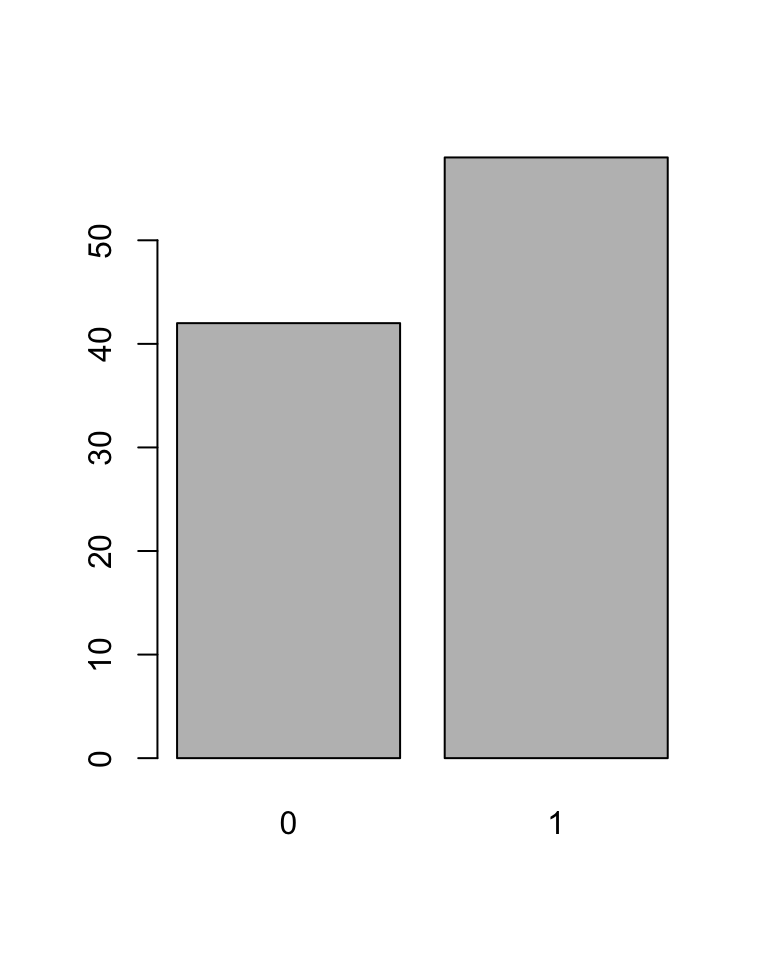Next we get more familiar with binomial distribution and some R functions by which we can evaluate probabilities of different outcomes in binomial experiments. These will later be a key to understand widely-used statistical concepts in medical research, such as P-values and confidence intervals.

Here is a mathematical formulation of binomial distribution. (Formulas are not needed in the exercises and concrete examples will follow below.)

Binomial distribution. Imagine we make $$n$$ trials (R calls this size) where each trial $$i=1,\ldots,n$$ results either in a success (1) or failure (0). We mark outcome of trial $$i$$ by $$x_i$$. So if trial $$i$$ was a success, $$x_i=1$$, and if trial $$i$$ was a failure, $$x_i=0$$. Assume that the probability of a success in each trial is $$p$$ (the same value for every trial). For example, above with a fair coin, $$p=0.5 = 50\%.$$ In this setting, we say that the total number of successes $$X = x_1+x_2+\ldots+x_n$$ follows Binomial distribution with size $$n$$ and success probability $$p$$, which we denote by $$X\sim\textrm{Bin}(n,p)$$.

From high school we (may) remember that the probability mass function for the binomial distribution is $f_\textrm{Bin}(k;n,p) = \textrm{Prob}(X=k) = {n \choose k} p^k (1-p)^{n-k}, \textrm{ for } k=0,\ldots,n.$ This probability mass function (“density function”, tiheysfunktio) tells for each value of $$k$$, what is the probability that we get exactly $$k$$ successes in Binomial distribution with parameters $$n$$ and $$p$$. It can be evaluated in R by dbinom(k, size = n, prob = p).

The cumulative distribution function (cdf, kertymafunktio) evaluates the probability that the number of successes is $$\leq k$$ and is given by summing the values of the probability mass function from 0 up to $$k$$: $F_{\textrm{Bin}}(k;n,p) = \textrm{Prob}(X \leq k) = \sum_{i=0}^k {n \choose i} p^i (1-p)^{n-i}, \textrm{ for } k=0,\ldots,n.$ This cdf can be evaluated in R by pbinom(k, size = n, prob = p).

#### Example 1.1.

Suppose that a treatment helps about 80% of cases. You are treating 10 patients. We want to evaluate how many of them will benefit? (This is a binomial experiment with $$\textrm{size}=10$$ and success probability $$p=0.8$$.)

1. In any one experiment (of treating 10 patients) the positive outcome can be anywhere between 0 and 10 patients. We want to learn statistical properties of this number of successes. Let’s start with an experimental approach and generate 100 experiments of the above mentioned setting of treating 10 patients and record the number of successes in each experiment. Make a barplot of the frequency table of the successes.
# To generate 100 binomial experiments, each of size = 10 and success prob = 0.8:
x = rbinom(100, size = 10, prob = 0.8)
# To plot the results with a barplot:
barplot(table(x), col = "limegreen") # 'col=' defines color, google "R colors" for available cols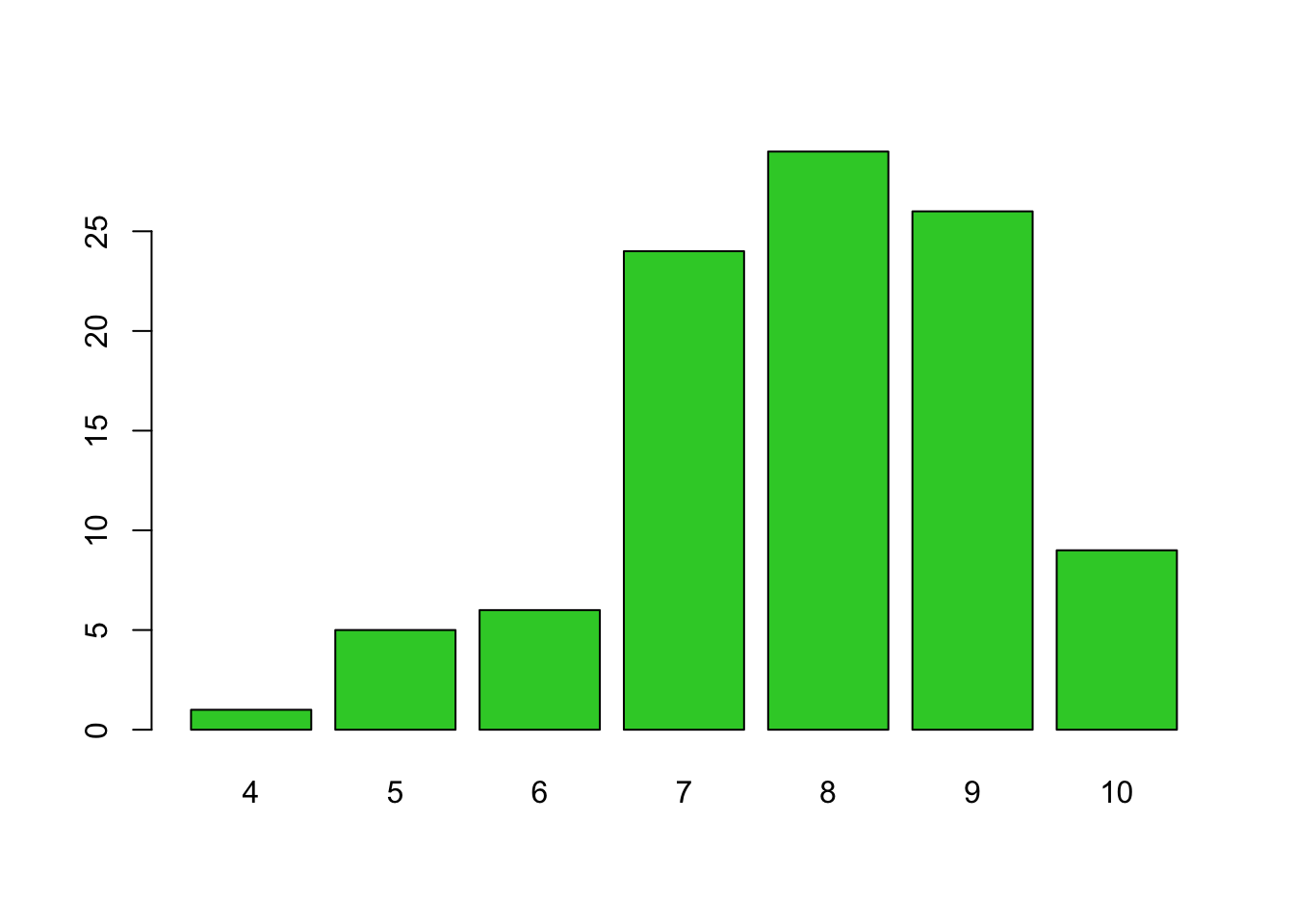It seems that values 8,9 and 7 are the most common ones, but also as small values as 4 occurred among the 100 random experiments. Values below 4 never happened in this series of 100 experiments so we conclude that they will happen very rarely when treating 10 patients and the average success rate is 80%.

1. What is the theoretical probability of getting exactly $$k=6$$ successes in one experiment? Is that value consistent with what you see in the empirical data above based on 100 experiments?
# What is the probability mass at value 6 of a binomial distribution
# with size = 10 and success probability = 0.8 for any one trial?
dbinom(6, size = 10, prob = 0.8)
##  0.08808038

From the barplot, it seems that the value 6 has appeared a bit over 5 times out of 100, suggesting a frequency a bit over 5%. Thus it seems consistent with the theoretical value of 8.8%. (And it would get more and more accurate as the number of experiments was increased from 100 to, say, 100,000.)

1. What is the probability of getting at most $$k=6$$ successes? Is that value consistent with what you see in the empirical data above based on 100 experiments?
# Let's see what is the cumulative probability mass at value 6 of a binomial distribution
# with size = 10 and success probability = 0.8 for any one trial:
pbinom(6, size = 10, prob = 0.8)
##  0.1208739

From the barplot, we sum up the counts that are at most 6: there are about 12 or so of them out of 100 observations. Thus result is consistent with the theoretical probability of 12.1% that value of successes is at most 6 in binomial experiment with size = 10 and prob = 0.8.

1. What is the probability of getting at least $$k=6$$ successes?

We’ll check the result in three different ways.

# pbinom( ) gives probability cumulated from 0 up to the given value
# Then 1 - pbinom( ) is the probability that is not included between 0 and the given value.
# Here we compute probability from 0 up to value 5 and then subtract that from 1:
1 - pbinom(5 , size = 10 , prob = 0.8) 
##  0.9672065
# We can also ask directly the upper tail of cumulative probability by 'lower = FALSE'
pbinom(5, size = 10 , prob = 0.8, lower = FALSE) #lower = FALSE -> gives the upper tail probability 
##  0.9672065
# To check, we can also simply sum up the probability mass given by dbinom applied to values 6,7,8,9,10:
sum(dbinom(6:10, size = 10, prob = 0.8)) 
##  0.9672065
1. If we want to find the cutpoint for the number of successes so that only 1% of the experiments gives a lower number of successes, we can use qbinom(q, size, prob) that evaluates the quantiles of the binomial distribution and set the level of the quantile to correspond to 1% = 0.01.
qbinom(0.01, size = 10, prob = 0.8)
##  5

This tells that the probability that the number of successes is < 5 is 1% or less in a binomial experiment with 10 trials and success probability of 0.8.

### Histograms

Let’s do many more experiments (say 10,000) and in each experiment consider how many successes we would have when treating 1000 patients and the success probability per each patient is still 0.80. The result from each experiment is now the number of successes in that experiment and can vary between 0 and 1000. The expected value is $$0.8\times 1000 = 800$$ for any one experiment.

n.experiments = 10000
n.trials = 1000
p = 0.8
x = rbinom(n = n.experiments, size = n.trials, prob = p)
# 10000 experiments, each with 1000 patients with 80% success probability for each patient

# Now we don't want to print all 10,000 values, but let's just have a peak at the first 10
x[1:10]
##   788 816 793 786 804 801 805 786 778 811
# Let's make summaries of data by summary()
# 1st Qu. = first quartile, 3rd Qu. = third quartile
summary(x)
##    Min. 1st Qu.  Median    Mean 3rd Qu.    Max.
##   747.0   792.0   800.0   800.1   809.0   846.0
# and by table() that shows all values and how many times they have been observed:
table(x)
## x
## 747 749 755 756 757 758 759 762 763 764 765 766 767 768 769 770 771 772 773 774
##   1   1   3   3   2   1   4   9   2   8   4  10  12  22  21  22  28  19  28  30
## 775 776 777 778 779 780 781 782 783 784 785 786 787 788 789 790 791 792 793 794
##  49  42  51  79  84  75  99 110 119 148 169 186 182 203 194 202 242 242 236 278
## 795 796 797 798 799 800 801 802 803 804 805 806 807 808 809 810 811 812 813 814
## 295 292 326 317 344 314 312 307 319 314 287 275 254 248 245 272 197 214 187 180
## 815 816 817 818 819 820 821 822 823 824 825 826 827 828 829 830 831 832 833 834
## 154 144 154 130 105  83  80  55  76  46  40  30  35  28  22  21  11   7  12   9
## 835 836 837 838 839 840 841 842 843 844 846
##  12   7   4   3   2   2   3   3   1   1   1
# and let's visualize by barplot:
barplot(table(x), col = "skyblue")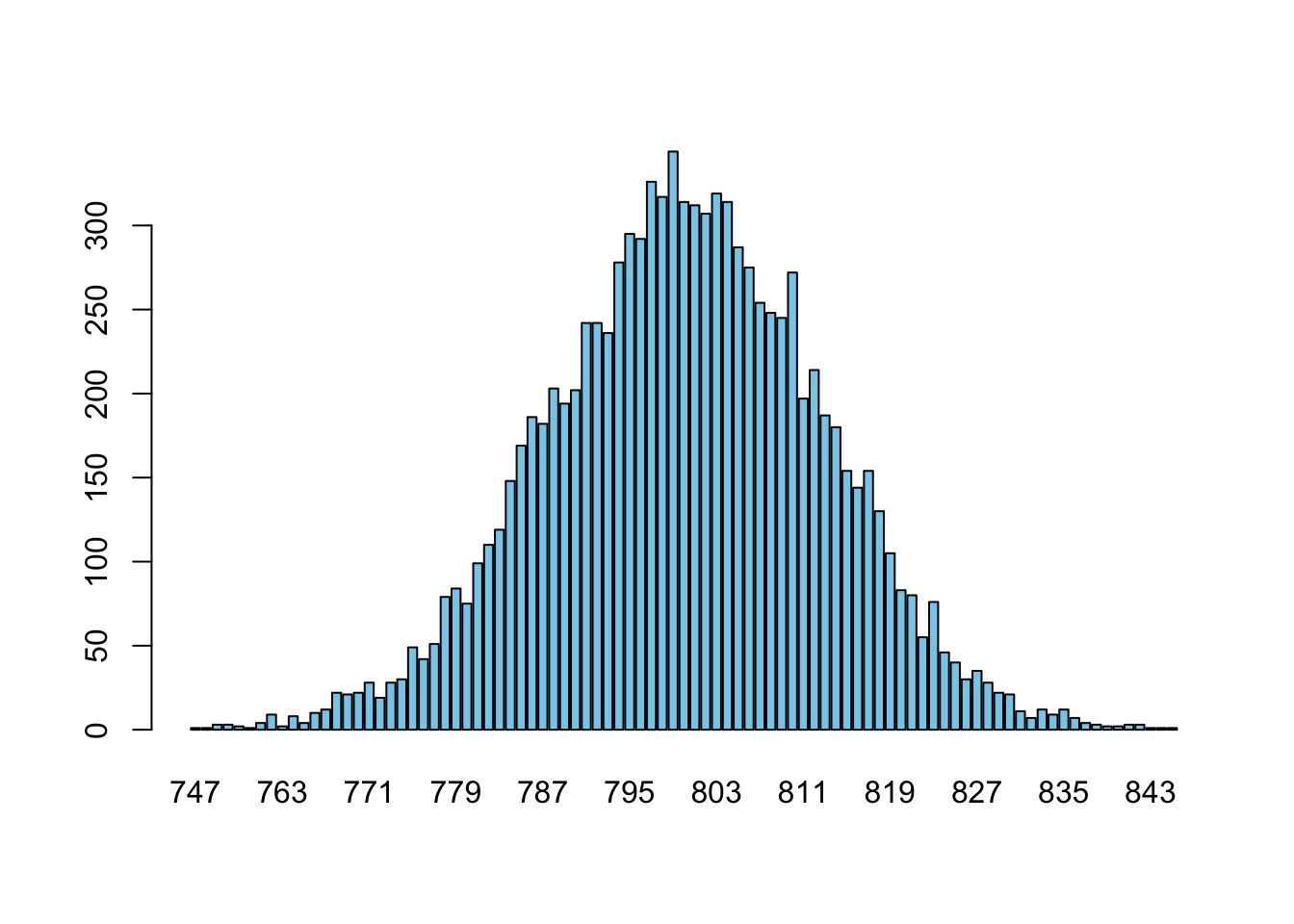Above barplot works fine, but if we would have, say, thousands of different observed outcome values, then we would not anymore want to plot a separate bar for each value but we would rather bin values near each other into a single bar. This is the idea of histogram, that shows the distribution by applying automatic binning of bars. In R, a histogram is produced by calling the hist( ) function.

hist(x, col = "steelblue")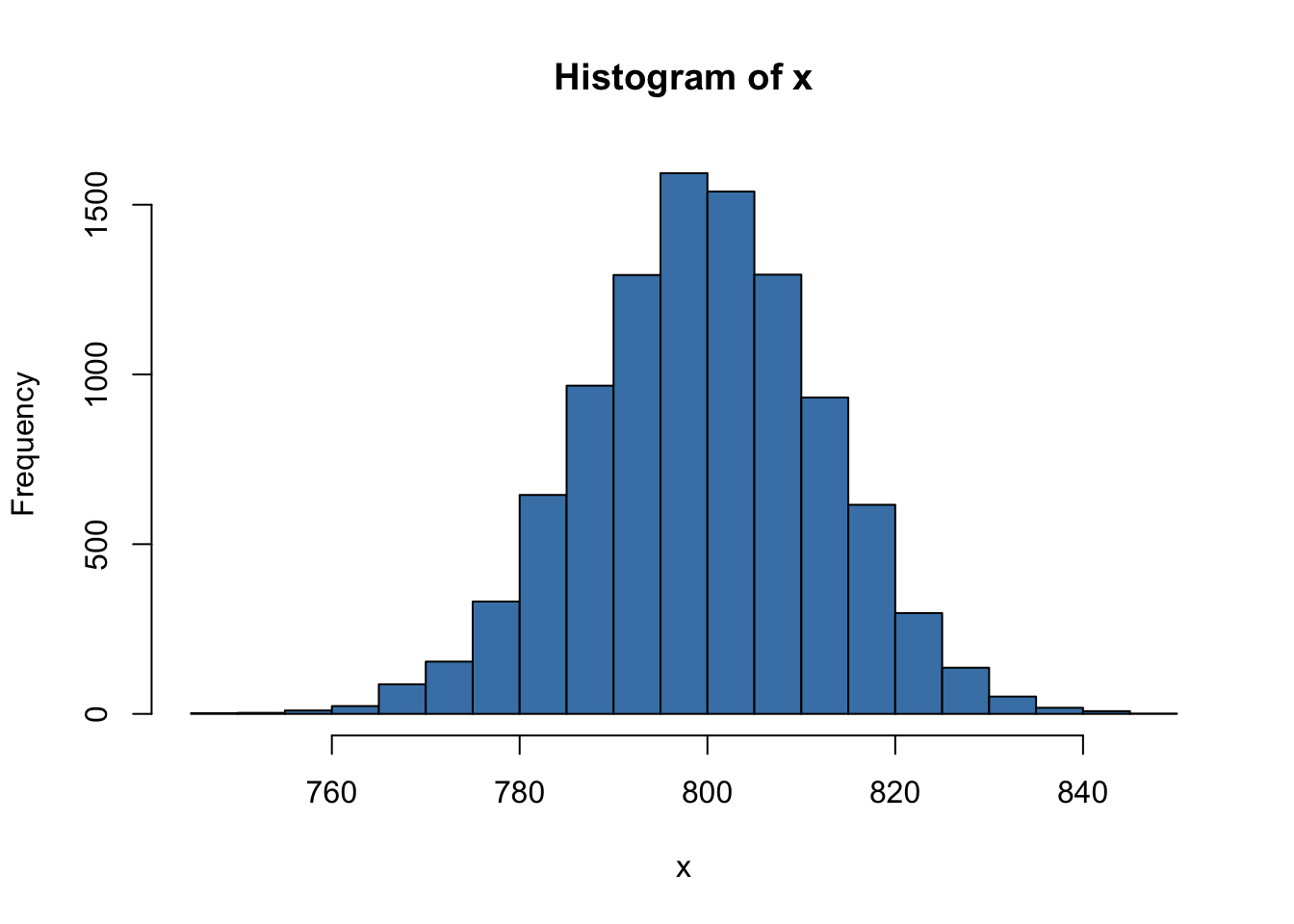We could also influence how many bins we want to have in the histogram by suggesting a number of bins via breaks parameter. Let’s suggest 10 bins, and compare the plot to the above plot that had about 20 bins. (Note that R doesn’t necessarily use exactly 10 bins, but chooses a “nice” value near the suggested value.)

hist(x, col = "dodgerblue", breaks = 10)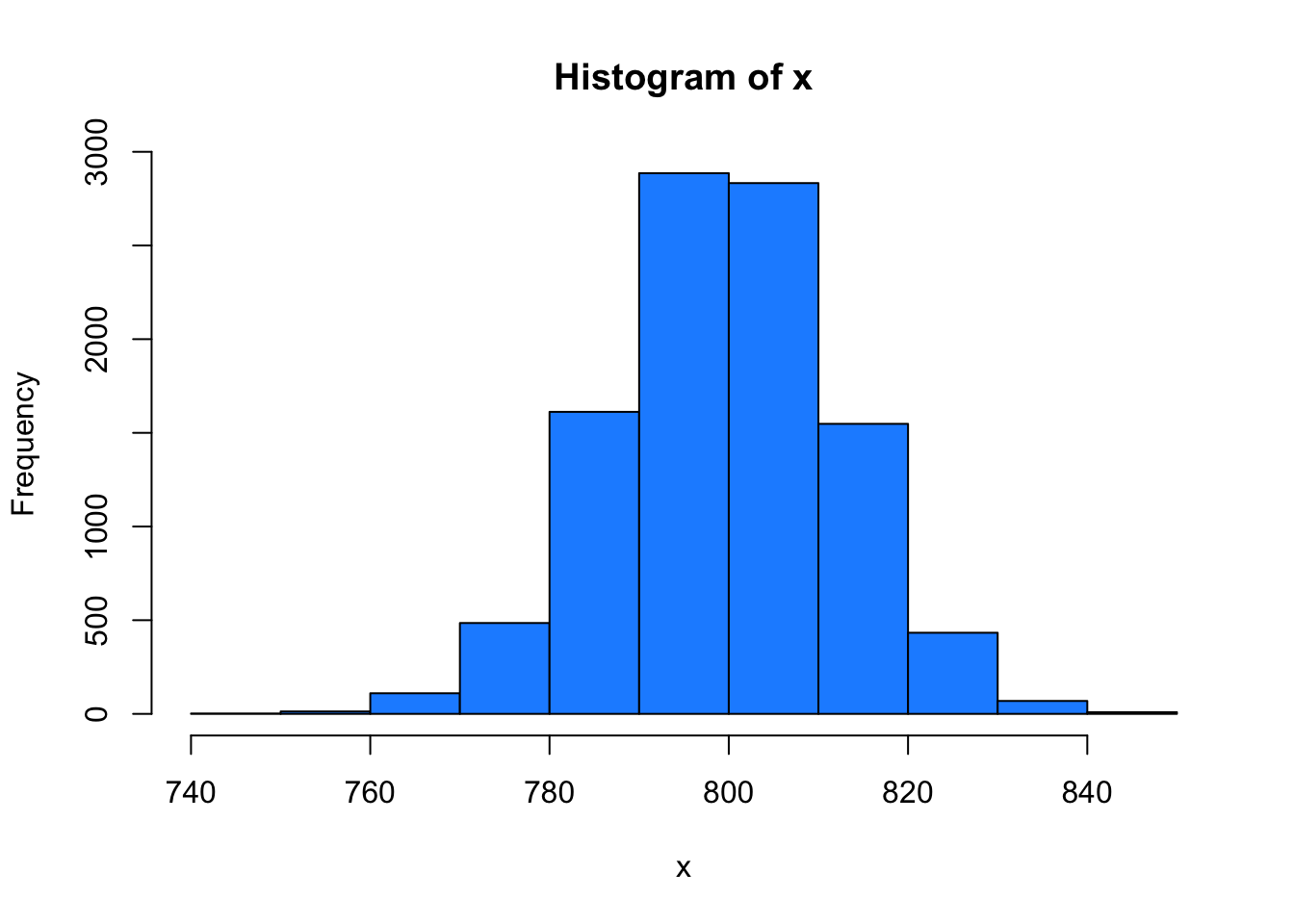As a conclusion from this experiment, with a simple R command we have, in a few seconds, “tossed a weighted coin” 10000x1000 = 10 million times. This ability to see how random variation behaves for different sample sizes and success probabilities will be crucial when we do justified conclusions about whether the observed results in, say, a clinical trial, could simply result from random variation, or whether some real differences between groups are likely to be present.

#### Summary of the four functions for binomial distribution

We have seen the use of four central R-functions related to binomial distribution.

1. First, with rbinom(1, 100, 0.5) we generated a random sample from Bin(100,0.5) (‘r’ for random).
2. Second, with dbinom(800, 1000, 0.8) we can compute the probability of observing exactly 800 successes when sampling a random variable from Bin(1000, 0.8) (‘d’ for density function, tiheysfunktio).
3. Third, with pbinom(800, 1000, 0.8) we can compute the cumulative probability of observing value at most 800 when sampling from Bin(1000, 0.8) (‘p’ for probability, kertymäfunktio).
4. The fourth function is qbinom(0.975, 1000, 0.8) that would tell the cutpoint to left of which 97.5% of the probability mass from Bin(1000,0.8) falls (‘q’ for quantile function, kvantiilifunktio).

For example, if we want to know that what are the values such that 2.5% of the distribution Bin(1000, 0.8) is to the left of the value, or to the right of the value, we would compute these cutpoints as

qbinom(c(0.025, 0.975), 1000, 0.8)
##  775 824

Thus, we have learned that 95% of the observations from Bin(1000, 0.8) remain between 775 and 824.

#### Example 1.2

1. Make a barplot of theoretical probability mass function of Bin(10, 0.5), i.e. binomial distribution with 10 trials and each trial has success probability of 0.5. Give some interpretation for this distribution in terms of patients and successful treatments.
# names.arg gives the names for the bars
# ylab and xlab give names for y-axis and x-axis for any plotting command
barplot( dbinom(0:10, 10, 0.5), names.arg = 0:10,
ylab = "probability", xlab = "successes", col = "red" )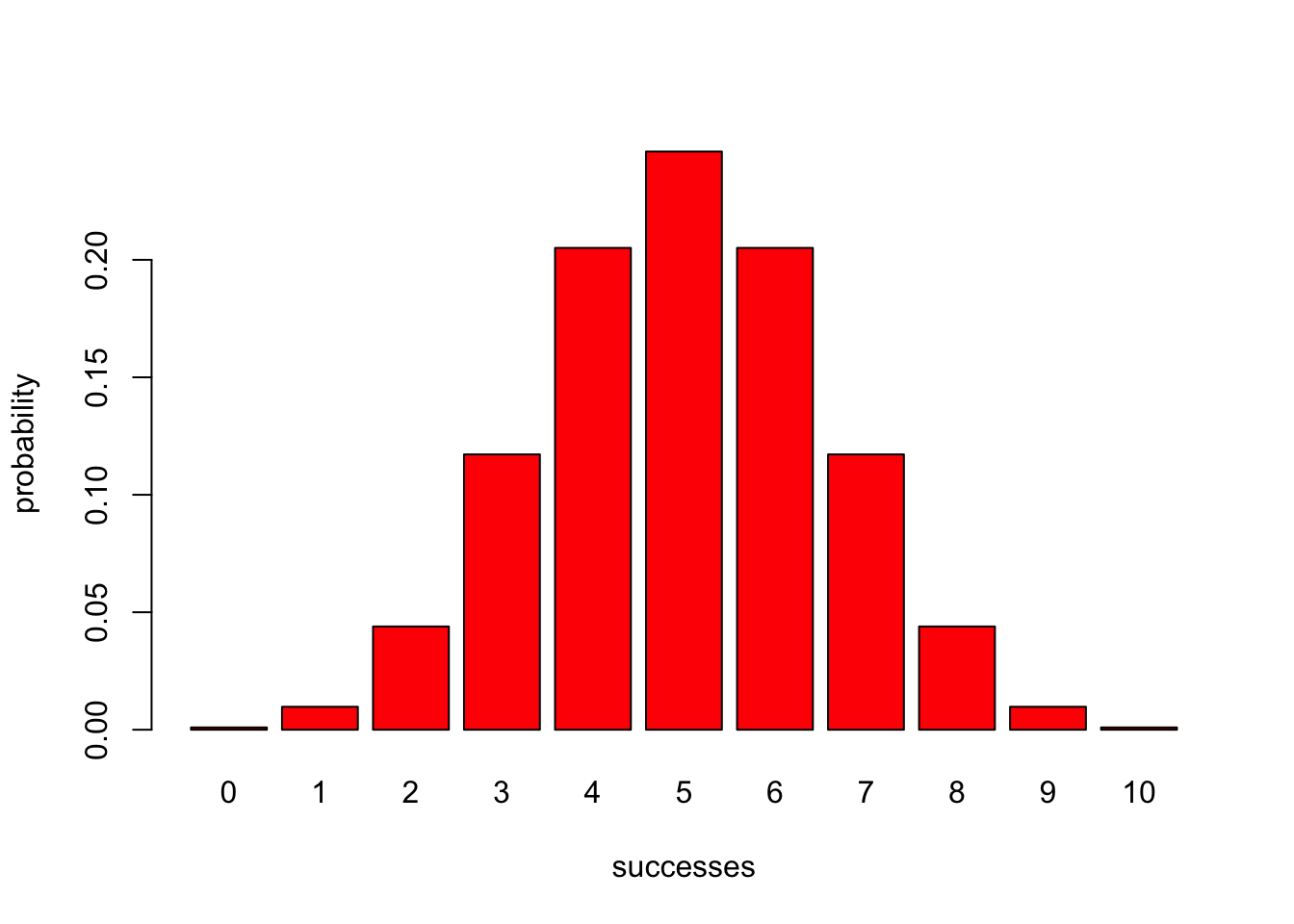For example, this distribution could describe how many patients out of 10 treated patients get better when the success rate of the treatment is 50%.

1. Estimate visually from the barplot what is the probability that you would get exactly 6 successes in 10 trials. Compute also the exact value using probability mass function and compare to your estimate.
dbinom(6, 10, 0.5)
##  0.2050781
1. Estimate visually from the barplot what is the probability that you would get more than 6 successes in 10 trials. Compute also the exact value using cumulative distribution function and compare to your estimate.
1 - pbinom(6, 10, 0.5)
##  0.171875
1. Estimate visually from the plot what would be a cutpoint at which the probability of the left tail is 10%. Use quantile function of the binomial distribution to check the exact value.

We see that the sum of bars from 0 to 2 remains below 10% while bar for 3 itself is > 10%. Thus the cutpoint asked seems to be 3, as verified by qbinom() function:

qbinom(0.1, 10, 0.5)
##  3